Practice M362K Final Exam

Originally Given Fall 1996

This exam consists of 7 problems, each worth 14 points. (You get 2 points for writing your name and ID#). Please read the ENTIRE test before attempting any problems, as you may find some of the later problems easier than the earlier ones.

Problem 1. Politics As Usual

It is a fact of politics that having control of the White House makes it harder to take control of Congress, and vice-versa. Since Clinton won the '96 presidential election, there is an 80% chance that the Republicans will keep control of Congress in '98. But if the Republicans keep control of Congress, then Al Gore will have a 60% chance of being elected president in 2000. If the Republicans don't keep control of Congress in '98, then Gore will only have a 30% chance of being elected in 2000.

Assuming, of course, that these estimates are accuratea) What is the probability that Al Gore will be elected president in the year 2000?

b) If Gore is elected in 2000, what is the probability that the Republicans held Congress in '98?

Problem 2. Don't Stub Your Toe!

Clumsy Carl gets injured a lot. He hurts himself an average of 0.2 times per day, and his injuries are described by a Poisson process.

a) What is the probability that Carl gets injured exactly twice today?

b) What is the probability that Carl gets injured no more than once this week?

c) Estimate the probability that Carl gets injured 80 or more times in 1997. [Give me a numerical answer, like 0.3424, and try not to forget the continuity correction!]

Problem 3. Means, Variances, and Moment Generating Functions

Let X be a discrete random variable that takes on the values -1, 0, and 1 with probabilities 0.1, 0.3, and 0.6, respectively.

a) Find the mean and variance of X.

b) Compute the moment generating function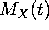.

c) Let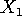and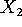be independent random variables, each with the same distribution as X. Let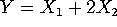. Find the mean, variance, and moment generating function of Y. [The formula for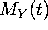is a little bit grungy. You do not have to simplify.]

Problem 4. Sample Spaces

Allan, Bob, Chris, David and Ed (whom you can abbreviate as A,B,C,D and E) play on the Podunk High School basketball team. At the end of the year, one or two of them will be chosen for the county all-star team.

a) Describe the sample space. [Either list all the possible outcomes, or give such a clear description in words that it is impossible to misunderstand you].

b) Now assume that all outcomes in the space are equally likely. (This is unrealistic, but hey, this is an exam question). What is the probability that Allan is chosen? What is the (conditional) probability of Bob being chosen given that Allan is chosen?

Problem 5. The Queen of Spades

In the game of Hearts, you are dealt 13 cards from a standard 52-card deck. It can be advantageous to be dealt the queen of spades, but only if you are also dealt several other spades. (Being dealt the queen by itself can be a disaster). For parts b) and c), you can leave your answer in a form involving binomial coefficients; you do not need to simplify further.

a) What is the probability of being dealt the queen of spades?

b) What is the probability of being dealt 4 spades, not counting the queen? (That is, for this part of the problem we don't care whether you get the queen or not. We just care how many of the other 12 spades you get).

c) What is the probability of being dealt the queen of spades and exactly 4 other spades?

Problem 6. Continuous random variables (2 pages!)

Let X and Y be continuous random variables, with the joint probability density function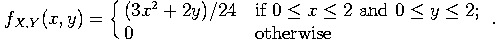a) Compute the cumulative distribution function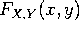, for x and y both between 0 and 2.

b) What is the probability that X <1?

c) Compute the marginal density functions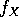and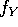.

Extra Credit: Compute the probability that X+2Y < 3.

Problem 7. The Acid Test

In a laboratory, chemistry students are told to mix 100 ml of distilled water with 50 ml of sulphuric acid. Of course, their measurements aren't exact. They measure the water with a mean of 100ml and a standard deviation of 4ml. They measure the acid with a mean of 50ml and a standard deviation of 3ml. The two measurements are independent, and are both described by normal distributions.

a) What is the probability of a given student measuring out at least 103 ml of water?

b) What is the probability of a given student measuring out at most 48.5 ml of acid?

c) What is the probability of a given student measuring out between 148ml and 157ml of liquid, total?